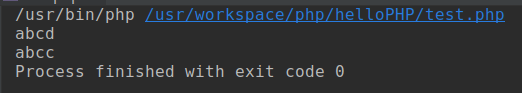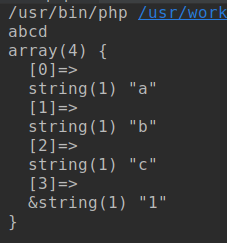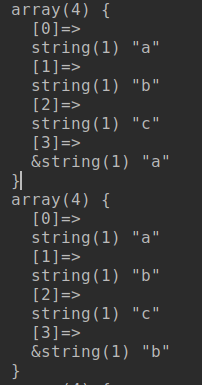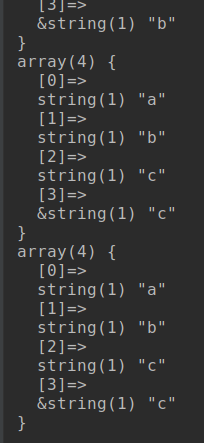# 问题

<?php
$arr = [ 'a', 'b', 'c', 'd', ]; foreach ($arr as &$each){ echo$each;
}
echo PHP_EOL;
foreach ($arr as$each){
echo $each; }  这段代码很简单, 输出数组的元素两次, 感觉会输出两次 abcd? 不好意思, 输出结果如下:是不是感觉很奇怪？我没有给数组赋值啊，数组的最后一个元素怎么在第二次循环的时候改变了呢？ # 问题分析 再来看下面一段修改过得代码： <?php$arr = [
'a', 'b',
'c', 'd',
];

foreach ($arr as &$each){
echo $each; } echo PHP_EOL;$each = '1';
var_dump($arr);是不是发现了什么？ 修改each变量会修改arr的最后一个元素, 这是为什么呢? 有过C语言使用经验的大概一看就明白是怎么回事了. 仔细看上面的foreach循环, each变量使用了&符号, 这个符号相当与c中的取址 php的foreach会在每次循环时,讲当前元素赋值给each, 然后进入循环体 当foreach遍历完成后, each变量没有释放而是指向了arr数组中的最后一个元素, 所以在后面给each赋值时, 其实改变的时arr数组的最后一个元素 到此, 流程已经明白了, 下面还原一下最开始的两次foreach过程: 在第一个foreach完成之后, 显然, each是指向数组的最后一个元素的, 下面进入第二个foreach: • 第一次遍历, 将arr赋值给each, 相当于arr=arr, 此时arr为: ['a', 'b', 'c', 'a'] • 第二次遍历, 将arr赋值给each, 相当于arr=arr, 此时arr为: ['a', 'b', 'c', 'b'] • 第三次遍历, 将arr赋值给each, 相当于arr=arr, 此时arr为: ['a', 'b', 'c', 'c'] • 第四次遍历, 将arr赋值给each, 相当于arr=arr, 此时arr为: ['a', 'b', 'c', 'c'] 分析的结果与之前输出的结果相同, 我们将第二次foreach的每次变化打印出来, 代码如下: <?php$arr = [
'a', 'b',
'c', 'd',
];

foreach ($arr as &$each){
}
foreach ($arr as$each){
var_dump(\$arr);
}posted @ 2019-07-14 16:57  烟草的香味  阅读(1353)  评论(0编辑  收藏  举报